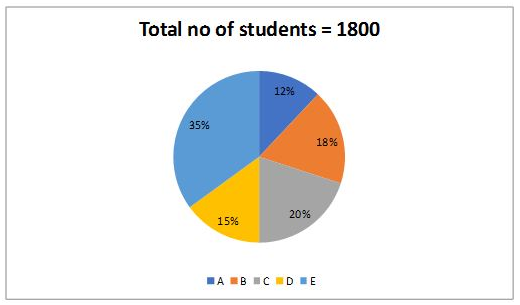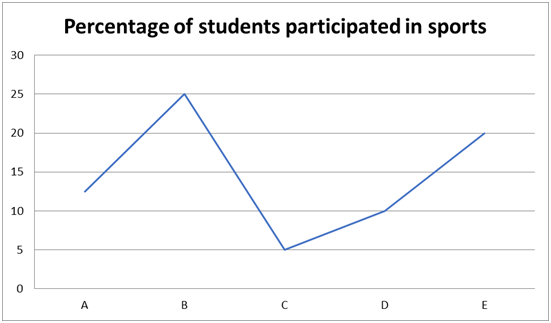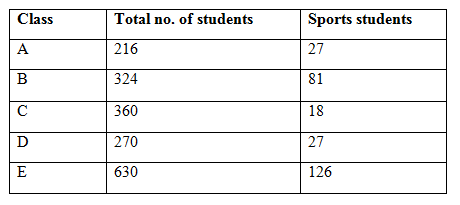# SBI Clerk Mains Quantitative Aptitude (Day-73)

Dear Aspirants, Our IBPS Guide team is providing new series of Quantitative Aptitude Questions for SBI Clerk Mains 2020 so the aspirants can practice it on a daily basis. These questions are framed by our skilled experts after understanding your needs thoroughly. Aspirants can practice these new series questions daily to familiarize with the exact exam pattern and make your preparation effective.

Start Quiz

Ensure Your Ability Before the ExamTake SBI Clerk 2020 Mains Free Mock Test

Data Interpretation

Directions (01 – 05): Study the following information carefully and answer the questions given below.

Pie chart shows the percentage of students in each class and line graph shows the percentage of students participated in sports.1) What is the ratio of the number of students from E to the students participated in sports from C & D?

A) 14: 1

B) 1: 14

C) 13: 21

D) 21: 13

E) None of these

2) What % is the total number of students participated in sports from all the classes together?

A) 15.5%

B) 17.8%

C) 20.3%

D) 13.5%

E) None of these

3) Students participating in sports from C & D together are how much % more/less than the no. of students in A?

A) 71.72%

B) 73.15%

C) 79.16%

D) 76.18%

E) None of these

4) What is the difference between the no. of students participating sports in A & B together and the total no. of students in D & E together?

A) 792

B) 996

C) 874

D) 926

E) None of these

5) In which of the following, the no. of students participating sports is higher?

A) A

B) E

C) B

D) C

E) None of these

Application Sums

6) 12 boys and 18 girls can complete a work in 6 days. 12 girls can complete the work in 18 days. 12 boys and 18 girls started the work 4 days after 4 boys left. In how many days, the remaining people will complete the work?

A) 12/5

B) 36/5

C) 48/5

D) 24/5

E) None of these

7) Express A leaves Chennai at 5 a.m. and reaches Mumbai at 9 a.m. another train Express B leaves Mumbai at 7 a.m. and reaches Chennai at 10:30 a.m. At what time they cross each other after 7 a.m.?

A) 56 min

B) 60 min

C) 14 min

D) 15 min

E) None of these

8) A man runs at a uniform speed and covers 36 km. Had the speed been 6 kmph less, it would have taken one more hour to complete the distance.

From the statement given in the above question which of the following can be determined.

A) Initial speed of the man

B) Initial time taken

C) Speed of the man in the latter case

D) Time taken by the man in the latter case

A) Only A

B) Only A & C

C) Only D

D) B & D

E) All of these

9) A boat takes 150 mins less to travel 40 km downstream than to travel the same distance upstream. If the speed of the stream is 4, what is the time taken to travel 25 km upstream?

A) 5/8 hr

B) 25/16 hr

C) 25/8 hr

D) 5/16 hr

E) None of these

10) Pipes A and B can fill the tank in 48/11 min but because of the drainage pipe C, it is being filled by 24/5 min. If C can drain off 15 l/min, what is the capacity of the tank?

A) 360 litres

B) 320 litres

C) 480 litres

D) 720 litres

E) None of these

Directions (01 – 05):Required ratio = 630: 45 => 126: 9 => 14: 1

Required percentage = 279/1800 * 100 = 15.5%

Required percentage = [(18 + 27) – 216]/216 * 100

= 171/216 * 100

= 79.16%

Required difference = 900 – 108 = 792

A – 27

B – 81

C – 18

D – 27

E – 126

Class E has maximum number of students participating in sports.

Directions (6-10):

(12b + 18g) * 6 = 12g * 18

12b + 18g = 36g

12b = 18g

b = 3/2g

(12b + 18g) * 4 + (8b +18g) * D = 12g * 18

b = 3/2g, so

36g * 4 + 30g * D = 12g * 18

24g + 5g * D = 36g

D = 12/5

s1 = x/4, s2 = 2x/7, t1 = y + 2, t2 = y

x/4 * (y + 2) + 2x/7 * (y) = x

(y + 2)/4 + 2y/7 = 1

y = 14/15

Required time = 14/15 * 60 = 56 min

36 = s * t —– 1

36 = (s – 6) * (t + 1) —– 2

From 1 and 2,

36/(s – 6) – 36/s = 1

s– 6s – 216 = 0

s = -12, 18

s = 18

t = 36/18 = 2

In latter case, s – 6 = 12

t + 1 = 3

Hence, we can find all from the given statement.

Speed of the boat = x

40/(x – 4) – 40/(x + 4) = 150/60 hr

x– 16 = 128

x = 12, -12

Now for 25 km, 25 = (12 –   4) * t

t = 25/8 hrs

1/A + 1/B = 11/48 —– 1

1/A + 1/B – 1/C = 5/24 —— 2

From 1 and 2,

1/C = 1/48

C drain off 15l/min,

48 * 15 = 720 litres

 Check Here to View SBI Clerk Mains 2020 Quantitative Aptitude Questions Day 72 Day 71 Day 70 Click Here for SBI Clerk 2020 – Detailed Exam Notification